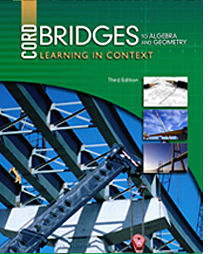# Bridges to Algebra and Geometry - 3rd Edition

## Chapter 9: Graphing on the Coordinate Plane

Some links are repeated for use with more than one lesson.

### 9.1 Coordinate Graphs

http://www.math.com/school/subject2/lessons/S2U4L1GL.html
This site defines coordinate planes and its components.

http://www.univie.ac.at/future.media/moe/galerie/zeich/zeich.html
NEW This site allows students to graph points and lines in the coordinate system (requires Java).

http://www.mathsisfun.com/data/cartesian-coordinates.html
NEW This page presents and practices the use of the Cartesian coordinate system.

### 9.2 Graphing Linear Equations

http://www.mathsisfun.com/data/straight_line_graph.html

https://www.desmos.com/calculator
NEW An online graphing calculator: simply type in your equations.

### 9.3 Slope

http://www.mathsisfun.com/geometry/slope.html
NEW This page defines the characteristics and formula for finding slope, with some practice problems.

http://www.coolmath.com/algebra/08-lines/05-finding-slope-line-from-graph-01.htm
NEW This site has a couple pages that show how to calculate the slope of a line graph.

### 9.4 Slope-Intercept Form

http://www.mathsisfun.com/equation_of_line.html
NEW This page presents and provides practice for defining lines when given the slope and intercept values, and finding those values for a given line graph.

### 9.5 Systems of Equations

http://www.purplemath.com/modules/systlin2.htm
NEW This site contains a couple pages that show the possibilities for solving a pair of linear equations graphically, and some of the limitations of a graphical method.

http://www.mathplanet.com/education/pre-algebra/graphing-and-functions/solve-systems-of-equations-by-graphing
NEW This site explains and provides a video tutorial of how to graphically solve a pair of linear equations.

### 9.6 Graphing Linear Inequalities

http://www.mathsisfun.com/algebra/graphing-linear-inequalities.html
NEW This page explains how to graph linear inequality, and provides some practice problems.

### 9.7 Relations and Functions

http://www.explorelearning.com/index.cfm?method=cResource.dspResourcesForCourse&CourseID=251
This site has examples and problems for students to solve that involve transformations.

http://www.regentsprep.org/Regents/math/geometry/GT1/Lreflect.htm
NEW This site presents an intuitive understanding of reflections. More advanced content is available, if desired.

http://www.mathsisfun.com/geometry/reflection.html
NEW This page provides some interactive learning and practice problems involving reflections.